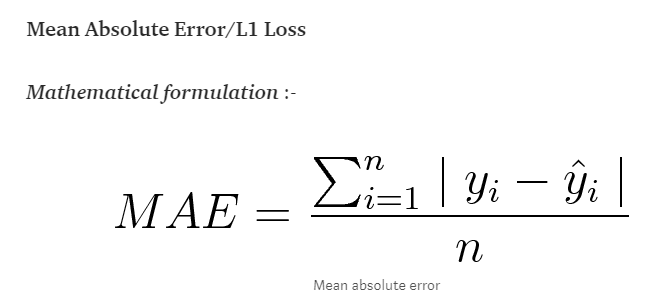# A simple but key question about back propagation chain rule in pytorch

``````from __future__ import print_function
import torch

xx = Variable(torch.Tensor(), requires_grad = True)
yy = 3*xx
zz = yy**2

yy.register_hook(print)
zz.register_hook(print)
tar = Variable(torch.Tensor(), requires_grad = False)
loss = zz-tar
loss.backward()
#normally here follows optimizer.step()
``````

Recently, I found that I did not even get straight with the real clear meaning of the back propagation and the optimizer.step()

So I tried the above code, what I felt confused is that no matter what the target is : the grad of yy, zz has the same value:

``````tar = Variable(torch.Tensor(), requires_grad = False)
tar = Variable(torch.Tensor(), requires_grad = False)
tar = Variable(torch.Tensor(), requires_grad = False)

``````

But these two sure change when xx change,

``````xx = Variable(torch.Tensor(), requires_grad = True)

and

``````

So I feel confuse that:
How could it possible that the grad of computation graph node has no relation to target, from my perspective, if the loss is larger, the grad shall become larger as well, so they would optimize more in the optimizer.step()

Will optimizer such as torch.optim.SGD() would add connection between loss and grad?

Thanks a lot for reading all above!

The derivative of your loss function wrt the parameters is independent of `tar`.
If you use a loss function like `loss = (zz-tar)**2`, you’ll see the behavior you are expecting.

PS: I’m not a huge fan of tagging people, as this might demotivate others to post a solution.thx, I would take your suggestion’

But as you can see, my above code is like MAE:So if use MAE, the grad of each variable has no relation with the final loss, why people still use it ? (since the grad of yi is constant)And so is cross entropy loss (since the grad of yi is tar/yy , not containing loss)

The solution to the MAE criterion gives you the median, while the solution to the MSE criterion gives you the mean. In the former case you’ll get a robust solution, which means that your solution is robust to outliers, but might have multiple minima. So even though your derivative is partially constant, it’s still a valid approach, at least for some problems. Note that you’ll still get different signs for predictions smaller or greater than your median. I have to admit I’ve never used the MAE criterion for a “real” problem, so I’m not sure how it’ll perform using a deep learning model.
Hastie et al. describe both criteria way better than I can possibly do it in Elements of Statistical Learning, page 18-20.

In the case of cross entropy loss, the derivative would be `prob_i - target_i`, where `prob_i` is the softmax output at position `i` and `target_i` is the target value at position `i`.
Here is a small manual example:

``````batch_size = 2
nb_classes = 5
target = torch.empty(batch_size, dtype=torch.long).random_(nb_classes)